# Understanding TypeScript Generics

For beginners to TypeScript it’s easy to be overwhelmed by concepts like generics and to not understand why they exist and what they are used for.

This article aims to explain why they exist and provide some simple examples to illustrate what makes them useful.

## Basic example

A fundamental concept in programming is DRY - Don’t Repeat Yourself. Abiding by this principle means writing code that isn’t full of needless repetition. You can abstract common functionality in to utility functions that are then shared across many files. Generics are simply a tool that allows you to apply this principle alongside the types you are implementing.

Let’s imagine we have a function called `reverse` that will take an array of data and reverse it:

``````const reverse = (data) => {
const temp = [];

for (let i = data.length - 1; i >= 0; i -= 1) {
temp.push(data[i]);
}

return temp;
}``````

This function works without an issue when it is compiled to JavaScript. Unfortunately, it also makes us lose type-safety in our TypeScript.

The problem with this function is that it allows a developer to reverse an array of anything. As such, what it returns can be an array of anything. TypeScript will infer this and in fact that’s exactly how it will type the function.

Hovering over the function definition in VSCode shows me that typescript has inferred `data` to be of type `any` and the return value of type `any[]`: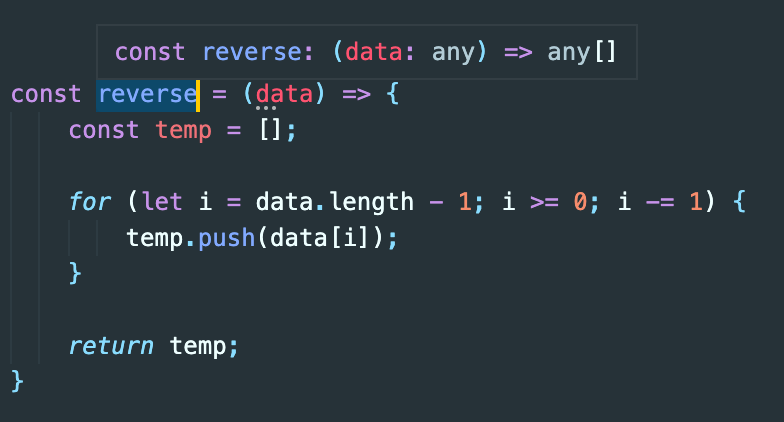This is an issue, because we will lose type-safety on anything that has been reversed by this function.

Look at the following example. We’ve declared a type `Person` and created an array of `Person` objects:

``````type Person = {
name: string;
age: number;
};

const people: Person[] = [
{
name: "Sean",
age: 28,
},
{
name: "Steve",
age: 42
}
];

const reverse = (data) => {
const temp = [];

for (let i = data.length - 1; i >= 0; i -= 1) {
temp.push(data[i]);
}

return temp;
};

// reversedPeople has lost its type of
// Person[] and is now type any[]
const reversedPeople = reverse(people);``````

Hovering over `reversedPeople` in our IDE we see it is no longer typed correctly: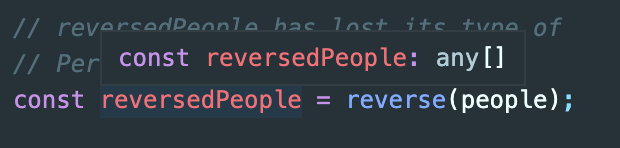By passing our array of people in to this function, we no longer have access to the type of the data anymore (which should still be `Person[]`). We are no longer protected against accessing a property of one of the objects in `reversedPeople` or trying to access a prototype method that doesn’t exist.

One solution to this problem would be to create multiple reverse functions for different types of data:

``````const reverseStringArrays = (data: string[]): string[] => {
const temp = [];

for (let i = data.length - 1; i >= 0; i -= 1) {
temp.push(data[i]);
}

return temp;
};

const reverseNumberArrays = (data: number[]): number[] => {
const temp = [];

for (let i = data.length - 1; i >= 0; i -= 1) {
temp.push(data[i]);
}

return temp;
};

const reversePersonArrays = (data: Person[]): Person[] => {
const temp = [];

for (let i = data.length - 1; i >= 0; i -= 1) {
temp.push(data[i]);
}

return temp;
};``````

Although this solves the problem, it means you will need to write a new utility function for pretty much every different piece of data you might wish to use this utility with. Not only is this time consuming, it also violates the DRY principle.

## Generics to the rescue

Luckily, this is a perfect opportunity to implement a generic type. We can use a `Type` or `T` variable which is a special variable specifically for types.

``````// adding generic type to a named function
const reverseStringArrays = function<T>(data: T[]): T[] {
const temp = [];

for (let i = data.length - 1; i >= 0; i -= 1) {
temp.push(data[i]);
}

return temp;
}

// adding generic type to an arrow function
const reverseStringArrays = <T>(data: T[]): T[] => {
const temp = [];

for (let i = data.length - 1; i >= 0; i -= 1) {
temp.push(data[i]);
}

return temp;
};``````

This syntax may look confusing at first because it’s the first major deviation from JavaScript syntax that new TypeScript developers will see, but it’s actually relatively straightforward.

The `<T>` before the parenthesis and arguments tells TypeScript what type is going to be passed in to the function. We then tell TypeScript that the function expects an argument which will be an array of `T` and the function will also return an array of `T` too.

TypeScript doesn’t really care what `T` actually is. All it knows is that whatever is passed to the function is the same type as what is returned.

This is why we use the name generic - the type will take the place of a wide range of types.

Using our example from above, we can now do the following:

``````type Person = {
name: string;
age: number;
};

const people: Person[] = [
{
name: "Sean",
age: 28,
},
{
name: "Steve",
age: 42
}
];

const reverse = <T>(data: T[]): T[] => {
const temp = [];

for (let i = data.length - 1; i >= 0; i -= 1) {
temp.push(data[i]);
}

return temp;
};

// Pass type Person as the generic type
const reversedPeople = reverse<Person>(people);``````

We have passed the type `Person` as a generic type to `reverse()`. TypeScript will now substitute `T` for `Person`. This means that the function has now been typed to accept an argument of `Person[]` and will also return `Person[]` too.

We can see that this works! In our IDE, hovering over `reversedPeople` now shows that type-safety has been restored: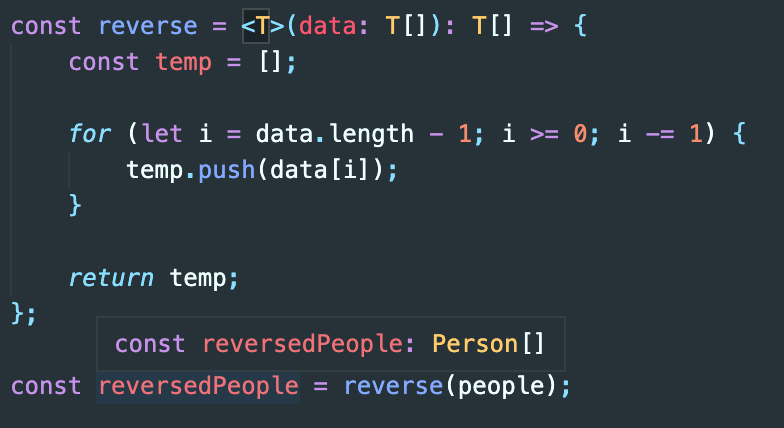We can now pass any generic type to this function and we will keep our type-safety:

``````const reversedNumbers = reverse<number>(arrayOfNumbers); // number[]

const reversedStrings = reverse<string>(arrayOfStrings); // string[]``````

If we try to pass something that doesn’t match the generic type, TypeScript will throw an error:

``````const mixedArray = [1, 2, 3, 'a', 'b', 'c'];

const reversedNumbers = reverse<number>(mixedArray); // Error

// Argument of type '(string | number)[]' is not assignable to parameter of type 'number[]'.
//  Type 'string | number' is not assignable to type 'number'.
//    Type 'string' is not assignable to type 'number'.``````

## Generic type extension

Consider a function that returns the cheapest item from an array of objects with a `cost` property:

``````const getCheapest = (data: Array<{ cost: number }>) => {
return data.sort((a, b) => a.cost - b.cost);
};``````

If we have an array of objects with type `House`, using this function will lose their type:

``````type House = {
address: string;
rooms: number;
cost: number
};

const houses: House[] = [
{ address: 'Westminster Street', cost: 10, rooms: 5 },
{ address: 'Trafalgar Street', cost: 20, rooms: 7 },
{ address: 'Downing Street', cost: 15, rooms: 11 }
];

const getCheapest = (data: Array<{ cost: number }>) => {
return data.sort((a, b) => a.cost - b.cost);
};

// cheapestHouse is typed as { cost: number }
const cheapestHouse = getCheapest(houses);``````

We can try to tell TypeScript that `cheapestHouse` is of type `House`:

``const cheapestHouse: House = getCheapest(houses);``

This won’t work. TypeScript will complain: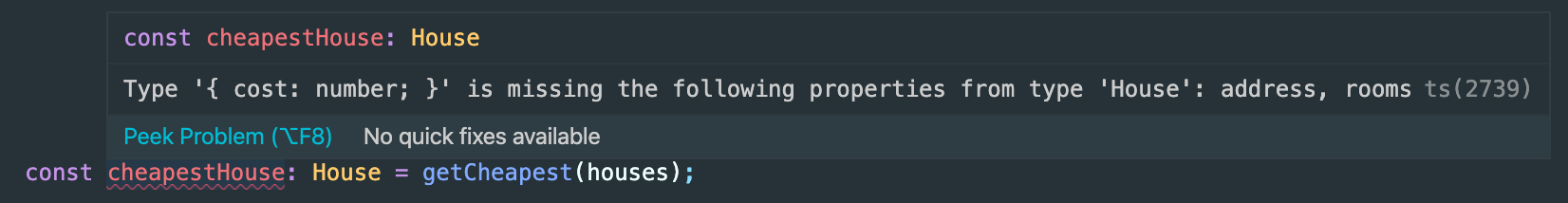We can’t just change the function to accept a `House` type, because then it won’t work with any other type of object that has a cost key:

``````type House = {
address: string;
rooms: number;
cost: number
};

type Car = {
make: string;
cc: number;
cost: number
};

const cars: Car[] = [
{ make: 'Mercedes', cost: 50, cc: 2500 },
{ make: 'BMW', cost: 70, cc: 3000 },
{ make: 'Audi', cost: 90, cc: 3500 }
];

const houses: House[] = [
{ address: 'Westminster Street', cost: 10, rooms: 5 },
{ address: 'Trafalgar Street', cost: 20, rooms: 7 },
{ address: 'Downing Street', cost: 15, rooms: 11 }
];

const getCheapest = (data: House[]) => {
return data.sort((a, b) => a.cost - b.cost);
};

const cheapestHouse = getCheapest(houses);
// Argument of type 'Car[]' is not assignable
// to parameter of type 'House[]'
const cheapestCar = getCheapest(cars); // ERROR``````

Again, generics to the rescue!

``````const getCheapest = <T extends { cost: number; }>(data: T[]): T => {
return data.sort((a, b) => a.cost - b.cost);
};``````

The extends keyword tells TypeScript that when we call the function `getCheapest`, we will pass a type `T` to it that will have at least one key `cost` which is of type number. TypeScript then knows that the argument will be an array of objects that can have any shape, as long as they also include a `cost` key. It also knows that the function needs to return one of these objects.

If we try to pass something that doesn’t have a `cost` key TypeScript will throw an error:

``````getCheapest(2); // ERROR
// Argument of type '2' is not assignable
// to parameter of type '{ cost: number; }[]'``````

Using the above example, we can now safely use this same function to get the cheapest `House` or `Car`:

``````const getCheapest = <T extends { cost: number; }>(data: T[]) => {
return data.sort((a, b) => a.cost - b.cost);
};

const cheapestHouse = getCheapest<House>(houses);
const cheapestCar = getCheapest<Car>(cars);``````

TypeScript is actually smart enough to be able to infer this without us having to explicitly pass the `House` or `Car` type to the function.

In my IDE - VSCode, the `cheapestHouse` and `cheapestCar` variables are already typed correctly without me passing their types to the function: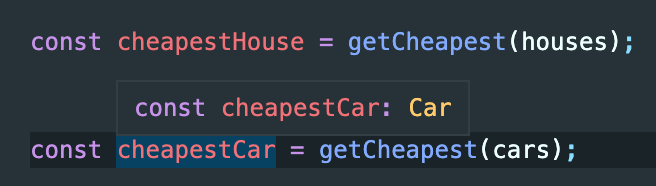We don’t just have to extend objects though, we can extend other types:

``````type ItemWithPrice = {
cost: number;
}

const getCheapest = <T extends ItemWithPrice>(data: T[]) => {
return data.sort((a, b) => a.cost - b.cost);
};``````

## Default Values

We can also set a default type for a generic. Take this example where we are creating a type called `Dictionary` for a regular JavaScript object:

``````type Dictionary<T=string> = {
[key: string]: T;
}``````

The type tells TypeScript that the object has a key of type `string` and a value of type `T`. By default `T` is assigned the value of `string`.

This means that we can create objects with string values without passing a generic type:

``````const person: Dictionary = { name: "Seán" };

// no need to pass string, because it is the default value
const person: Dictionary<string> = { name: "Seán" };``````

If we were to use a value of `number` however, we would need to pass that type as the generic:

``````const building: Dictionary<number> = { age: 101 };

// Type 'number' is not assignable to type 'string'
const building: Dictionary = { age: 101 }; // ERROR``````

## Multiple Generics

You are not limited to using just one generic variable. Consider this function that allows us to grab a key from an object:

``````const getObjectKey = <T, K extends keyof T>(object: T, keyToGrab: K): T[K] => {
return object[keyToGrab];
}

getObjectKey({ name : 'hello' }, 'name');

getObjectKey({ name: 'hello' }, 'age'); // ERROR
// Argument of type '"age"' is not assignable to parameter of type '"name"'``````

This looks complex, but it’s quite straightforward. We pass an object which replaces the type `T`. We then pass a string which replaces type `K`. `K` has been typed as `K extends keyof T` which means that K must be one of the keys within the object `T`.

Now, when we use the function TypeScript will not allow us to try and grab keys from the object that do not exist.

## Summary

Hopefully this article has helped you understand some of the basic use-cases for generics. They are an extremely useful tool in the TypeScript toolbox. If this helped you or you want to suggest any other topics, feel free to tweet me.